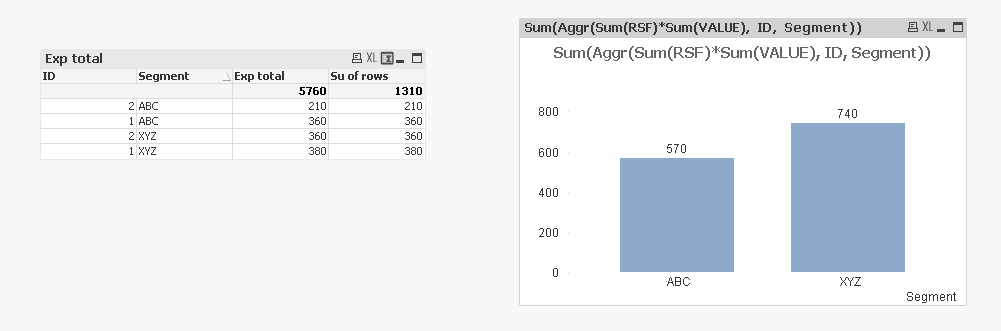# New to QlikView

Discussion board where members can get started with QlikView.

Announcements
Modernize Your QlikView Deployment webinar, Nov. 3rd. REGISTER
cancel
Showing results for
Did you mean:
HighlightedCreator

## Sum of Rows vs Expression Total.

Hi ,

I have below expression in the bar chart.

My dimension is Called Type.

=(If(GetFieldSelections(_BTUnitOfMeasure)='SQF',

(Sum([RSF])),

(Sum([RSF])))  * SUM(Value)

I am not getting the desired number,So tried with the straight table.Here I get different number with Expression total and sum of rows.

How can I get the same with both options.

Thank you,

1 Solution

Accepted Solutions
HighlightedMVP

May be you want this

Sum(Aggr(Sum(RSF)*Sum(VALUE), ID, Segment))7 Replies
HighlightedMVP

What are you getting and what was expected? Do you have a sample or images to show?

HighlightedMVP

May be try this

=If(GetFieldSelections(_BTUnitOfMeasure) = 'SQF', Sum([RSF]), Sum([RSF])  * Sum(Value))

HighlightedCreator

Hi Sunny...Thank you.

I have attached 2 files...QVW and excel.

How can I get sum of rows number in bar char ?

HighlightedCreator

HighlightedMVP

May be you want this

Sum(Aggr(Sum(RSF)*Sum(VALUE), ID, Segment))HighlightedCreator II

Hi,

Can you tell us which output your trying to get in the excel.

HighlightedCreator

Awesomeness....Thank you.